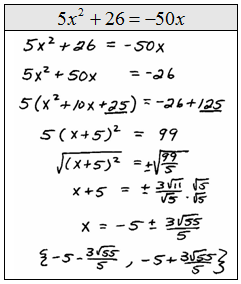## Pages

### Completing the Square

Solving by factoring and extracting the square roots are techniques that only work for very special quadratic equations. In this section, we will develop a method that will solve any quadratic equation. This technique will be used to develop the quadratic formula.is to be a perfect square then the constant term must equal 16. In this case, we can see thatTo know 16 was the number needed to complete the square we divided our middle term B = 8 by 2 to get 4 then squared that to obtain 16.

Complete the square then factor.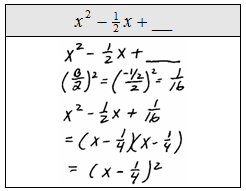The leading coefficient must be 1 if this method is to work. If it is not equal to 1 we could always factor the coefficient out first as in the next examples.

Determine the constant that completes the square.Now that you know how to complete the square for a quadratic expression you are ready to solve any quadratic equation. Notice that the next example does not factor, so we will solve it by completing the square.

Solve by completing the square:Step 1: Add or subtract the constant term to the other side.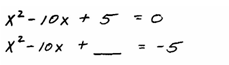Step 2: Add (b/2)^2 to both sides. Here B = −10 so (−10/2)^2 = (−5)^2 = 25Step 3: Factor and then apply the square root property.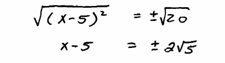Step 4: Solve as usual.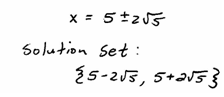Tip: Be sure to add the “magic number,”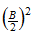to both sides so that the equality is retained.

Solve by extracting roots.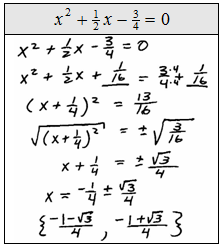So far we have seen examples where the leading coefficients are 1. But if this technique is to be useful for solving quadratic equations of the formwe must address the case where a is a number other than 1.

Solve by completing the square.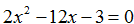Extra Step: Factor out the leading coefficient.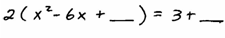Here B = −6 so (B/2)^2 = (−6/2)^2 = (−3)^2 = 9. Caution! Adding 9 inside the parentheses is equivalent to adding 18 here.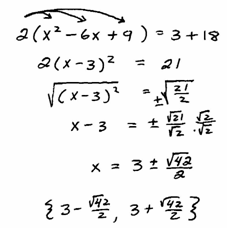Solve.